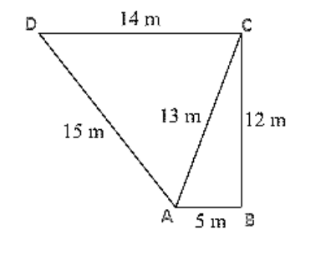# The sides of a quadrilateral, taken in order as 5 m, 12 m, 14 m, 15 m respectively,

Question:

The sides of a quadrilateral, taken in order as 5 m, 12 m, 14 m, 15 m respectively, and the angle contained by first two sides is a right angle. Find its area.Solution:

Given that the sides of the quadrilateral are

AB = 5 m, BC = 12 m, CD =14 m and DA = 15 m

Join AC

Now area of triangle ABC = ½ × AB × BC

= 1/2× 5 × 12

$=30 \mathrm{~m}^{2}$

In triangle ABC, By applying Pythagoras theorem

$A C^{2}=A B^{2}+B C^{2}$

$A C=\sqrt{5^{2}+12^{2}}$

AC = 13 m

Perimeter = 2s = AD + DC + AC

2s = 15 m + 14 m + 13 m

s = 21 m

By using Heron's Formula,

Area of the triangle PSR $=\sqrt{s \times(s-a) \times(s-b) \times(s-c)}$

$=\sqrt{21 \times(21-15) \times(21-14) \times(21-13)}$

$=84 \mathrm{~m}^{2}$

Area of quadrilateral ABCD = Area of triangle ABC + Area of triangle ADC

$=(30+84) m^{2}$

$=114 \mathrm{~m}^{2}$